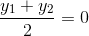# Circle and Chords intersected by x-axis

• AGNuke

#### AGNuke

Gold Member
Let a circle be given by 2x (x-a) + y(2y-b) = 0; (a≠0, b≠0).

Find the condition on a and b if two chords, each bisected by the x-axis, can be drawn to the circle from (a, b/2)

My attempt in this question is not quite relevant at this moment. I just found that (a,b/2) will lie on circle and the equation of chord being b(x-p) - 2y(a-p) = 0; where (p,0) is the midpoint of a chord.

Further than that, I have no idea what to do... :|

If the x-axis bisects the chord, what is the y-coordinate of both of the other endpoints?

One point of the chord, which is present on the circle as well is (a, b/2), which is given, other than that, no relevant info is given.

I'm trying to solve the question in due time and I hope I can solve this question in my second attempt, using entirely new approach.

My point is: The y coordinate of the point midway between (x1, y1) and (x2, y2) is given by $\displaystyle\frac{y_1+y_2}{2}\,.$

If $\displaystyle\frac{y_1+y_2}{2}=0$ (The y coordinate for any point on the x-axis is zero.), then if y1 = b/2, what is y2 ?

That's the problem, it is not given. But from section formula, it is obvious that it is -b/2

You don't need any formulas! If $(y_1+ y_2)/2= 0$ and $y_1= b/2$ then $(b/2+ y_2)/2= 0$ so, multiplying through by 2, $b/2+ y_2= 0$ and then $y_2= -b/2.$

But isn'tis a Section formula itself?

I don't know what "section" you mean. I would take "$(y_1+ y_2)/2= 0$" as coming from the given information that the x-axis bisects the chord.

Section Formula? To find out the point dividing the distance between two co-ordinates in a fixed ratio either internally or externally.